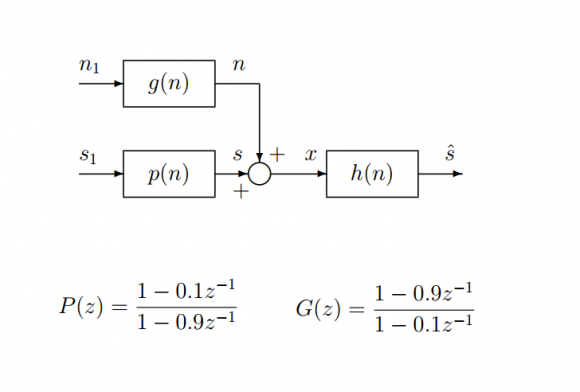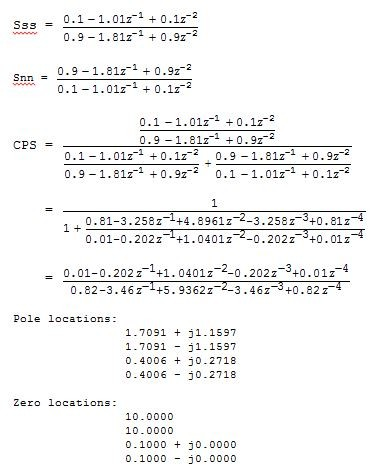## IIR non-causal Wiener filter solution giving unstable filterStarted by 7 years ago4 replieslatest reply 7 years ago409 views

Hello my fellow DSPers!

I was hoping someone could shed some light on a part of an assignment I have for a dsp module, I've been stuck on this particular part for two weeks now and can't see what I'm doing wrong.

I'll explain what I have so far,

If you refer to the image below you can see the system. h(n) is the Wiener filter. I have to come up with the optimum Wiener filter and then implement it in matlab for the 3 types of solution, FIR, IIR Causal and IIR non Causal. FIR was fine, but the IIR non-causal part I am having problems with.

n1 AND s1 are gaussian noise 0 mean unity Variance.

The transfer functions of these can be seen below. n is the noise to our signal s and these make our observed signal x. We are trying to get the minimum square error between s^  and s.

For the IIR non causal case the optimum Wiener filter can be described as the

Cross Power Spectral Density of s with x  over the spectral power density of x with x.  So Ssx/Sxx.

This can be re written as Sss/(Sss+Snn).   Since many things aren't correlated.

Spectral Power density of s can be written as  Sss=P(z)P(z^-1).  and Snn can be written as Snn=G(z)G(z^-1).    When I sub these answers into the equation  Sss/(Sss+Snn) for the optimum filter I get zeros at 0.1 and 10  and poles at 0.4006+0.272i, 0.4006-0.272i, 1.709-1.16i, 1.709+1.16i .

This has non stable response and it can't be implemented. It goes against what a Wiener Filter is so I think I made a mistake somewhere.

I hope this isn't too much hassle for someone to have a quick gander and see if they spot something, experience helps a lot in dsp :) ,

Thanks a lot, David.[ - ]Go back and review the relationship between causality and stability.

A rough definition of stability for an IIR filter is that all of its modes diminish to zero as time goes to infinity.  "Mode" in this case pretty much means "response of a pole".

For a causal filter, this means that each pole (in the z domain) must have an absolute value strictly less than one, because d^n goes to zero as n goes to positive infinity if and only if |d| < 1.

BUT!

For a non-causal filter, time goes backwards.  Stability is determined if each pole's response goes to zero as time gets more and more negative.  For this to happen, the pole's absolute value must be strictly greater than one -- which is exactly the indicator of instability in a causal filter.

You should review your material up to this point -- if you're need to implement a "non-causal" filter (of any sort), then you are in one of two situations:

1. You're working on "offline" data
2. You're screwed

If your situation is 2, then change the game.

If your situation is 1, then make a time-reversed version of the non-causal filter, and run it "in reverse" over the data.  This is a fairly standard thing to do in offline data analysis.

[ - ]Fantasic,

I'll give that a shot, I am indeed working on offline data.

I didn't know that about stability for a non-causal filter :D .

Thanks for the fast reply and best wishes.

[ - ]I dimly recall that Matlab has a one-step filter that does this -- it finds all the poles and zeros, puts the unstable one into the "non-causal" filter, runs the causal filter forward and the "non-causal" one backwards, and you're done.

But I use Scilab, so I can't tell you more.  It may be worth a bit of spelunking in the help files.

OTOH, if you're a student, it's probably worth making sure that you know how to do it by hand, to deepen your knowledge of signal processing theory.

[ - ]